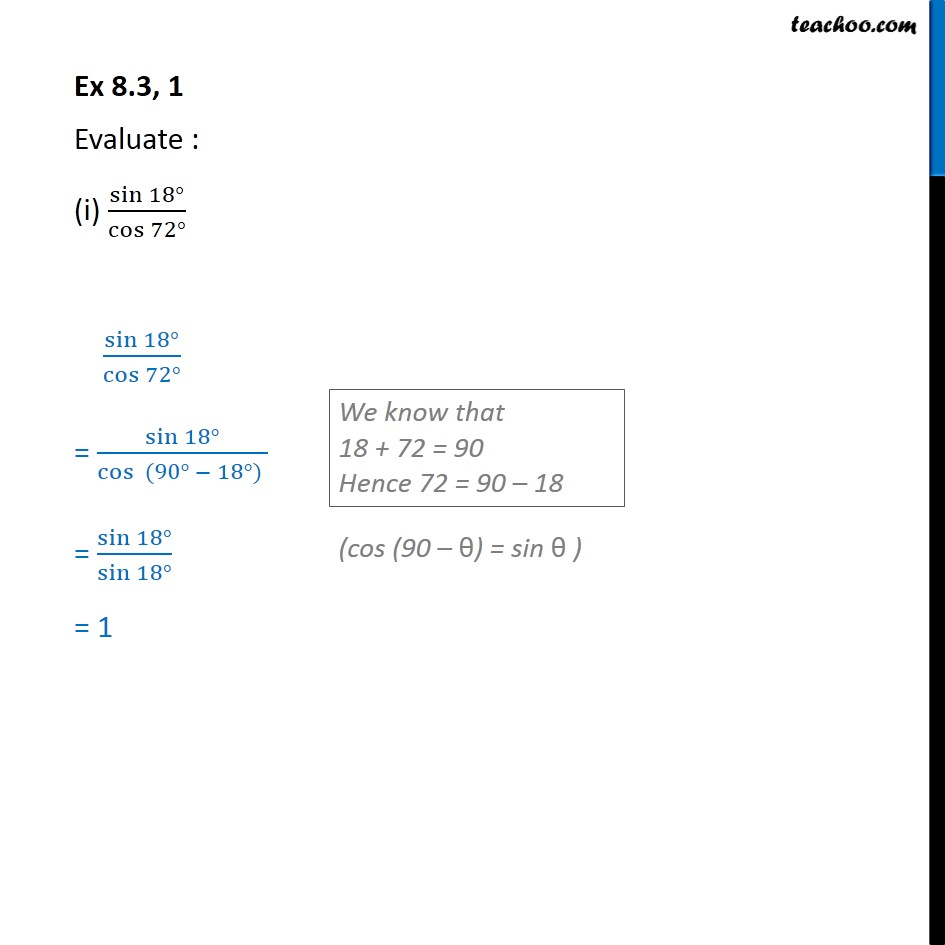1. Chapter 8 Class 10 Introduction to Trignometry (Term 1)
2. Concept wise
3. Trignometric ratios of complementry angles

Transcript

Ex 8.3, 1 Evaluate : (i) sin 18 /cos 72 sin 18 /cos 72 = sin 18 /cos (90 18 ) = sin 18 /(sin 18 ) = 1

Trignometric ratios of complementry angles